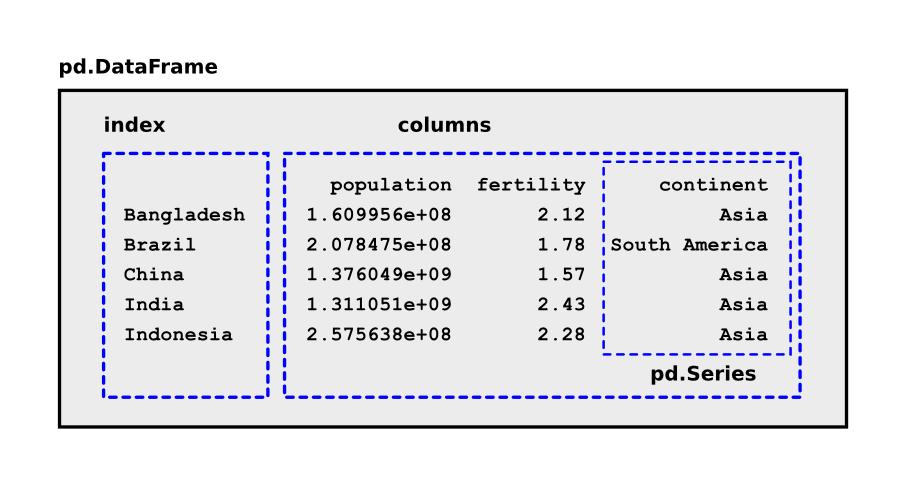# Analyzing tabular data with pandas

featuring the human proteome

## pandas

Pandas is the de-facto standard to read, analyze and visualize tabular data from CSV files, Excel tables and many more.

The main data structure for tables is called DataFrame:### Installing pandas

``````pip install pandas
pip install matplotlib
``````

## Your Task

1. Make sure you have the file `human_proteome.csv`.
2. Execute the paragraphs below in an interactive Python console.
3. Find out what each code segment does.

### Exercise 1

``````import pandas as pd
import pylab as plt

df = pd.read_csv('human_proteome.csv', index_col=0)
df.head()
``````

### Exercise 2

``````df['name']
df[['name', 'length']]
``````

### Exercise 3

``````df.sum()
``````

### Exercise 4

``````df['W'].mean()
df['W'].std()
``````

### Exercise 5

``````df.describe()
``````

### Exercise 6

``````df[df['length'] > 5000]
``````

### Exercise 7

``````df.sort_values('length', ascending=False).head(10)
``````

### Exercise 8

``````df['H_percent'] = df['H'] / df['length']
df.sort_values('H_percent', ascending=False).head(20)
``````

### Exercise 9

``````def match_name(name, query):
return query in name

hemo = df['name'].apply(match_name, args=["Hemoglobin"])
df[hemo]
``````

### Exercise 10

``````df.plot.scatter('K', 'R')
plt.axis([0, 1000, 0, 500])
plt.savefig('scatterplot.png')
``````

### Exercise 11

``````plt.figure()
short = df[df['length'] < 1000]
short['length'].hist(bins=50)
plt.savefig('lengths.png')
``````